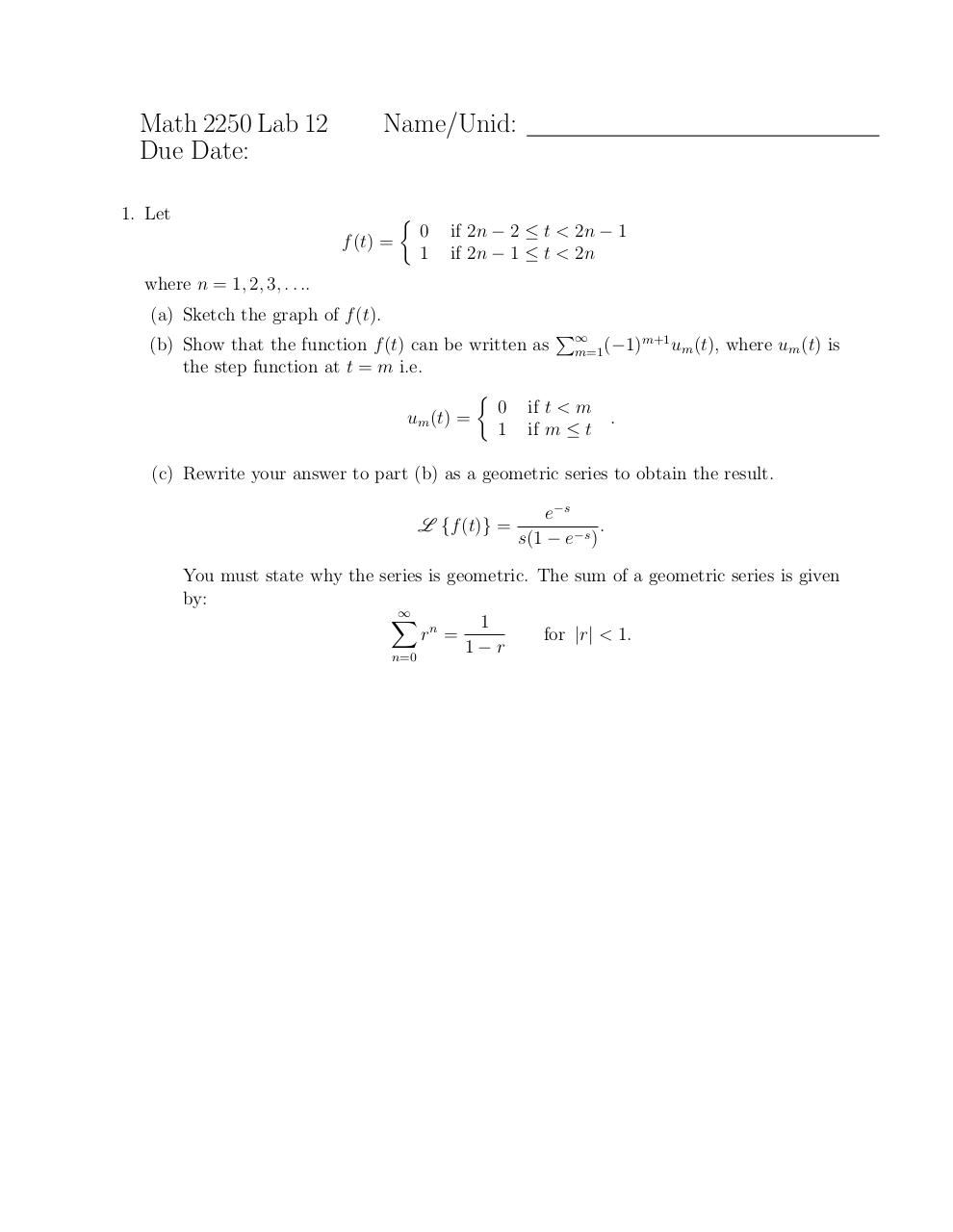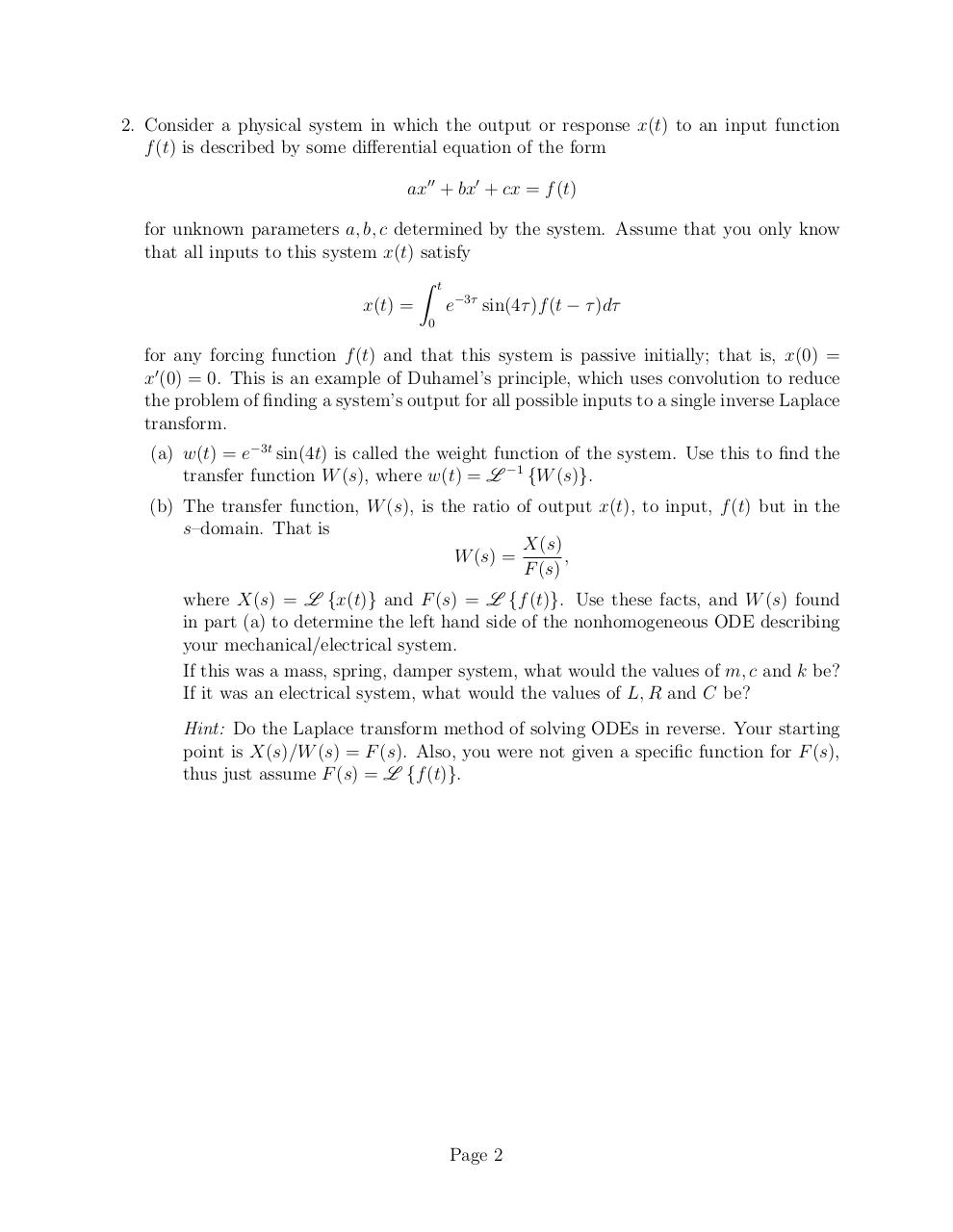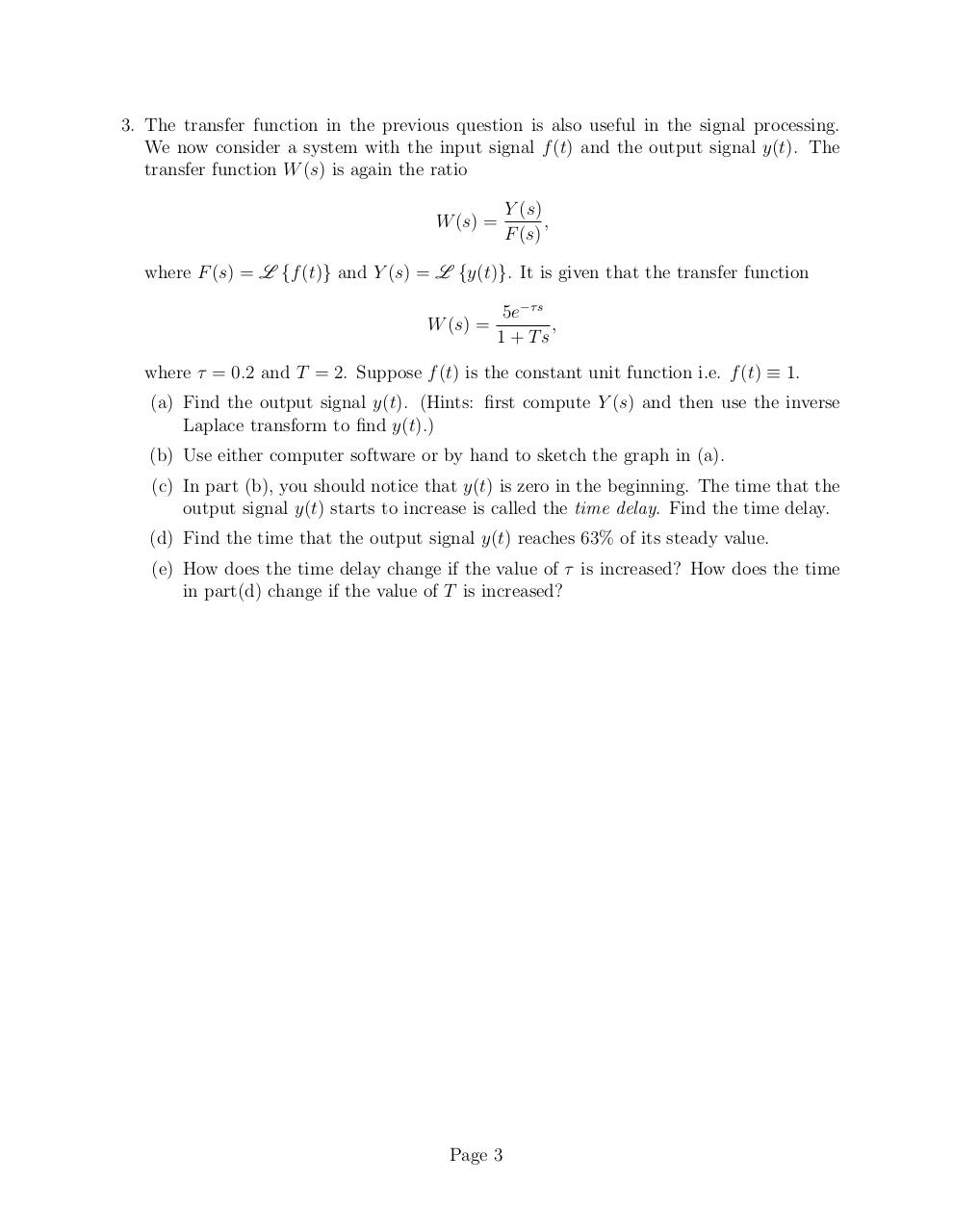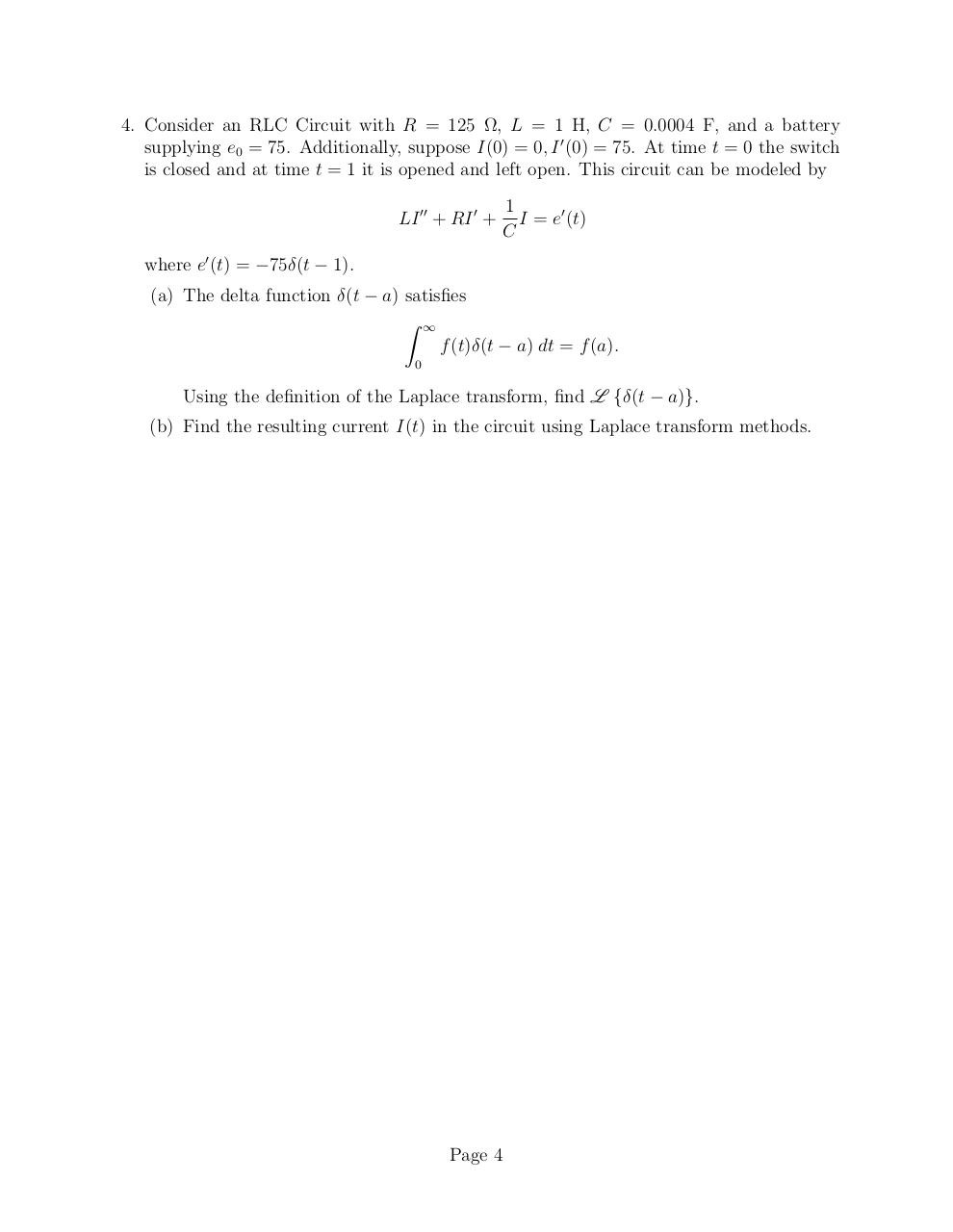# Lab12 HD .pdf

### File information

Original filename: Lab12_HD.pdf

This PDF 1.5 document has been generated by TeX / MiKTeX pdfTeX-1.40.14, and has been sent on pdf-archive.com on 02/12/2014 at 15:16, from IP address 76.27.x.x. The current document download page has been viewed 485 times.
File size: 110 KB (4 pages).
Privacy: public file

Lab12_HD.pdf (PDF, 110 KB)

### Document preview

Math 2250 Lab 12
Due Date:

Name/Unid:

1. Let


f (t) =

0
1

if 2n − 2 ≤ t &lt; 2n − 1
if 2n − 1 ≤ t &lt; 2n

where n = 1, 2, 3, . . ..
(a) Sketch the graph of f (t).
P
m+1
(b) Show that the function f (t) can be written as ∞
um (t), where um (t) is
m=1 (−1)
the step function at t = m i.e.

0 if t &lt; m
um (t) =
.
1 if m ≤ t
(c) Rewrite your answer to part (b) as a geometric series to obtain the result.
L {f (t)} =

e−s
.
s(1 − e−s )

You must state why the series is geometric. The sum of a geometric series is given
by:

X
1
rn =
for |r| &lt; 1.
1

r
n=0

2. Consider a physical system in which the output or response x(t) to an input function
f (t) is described by some differential equation of the form
ax00 + bx0 + cx = f (t)
for unknown parameters a, b, c determined by the system. Assume that you only know
that all inputs to this system x(t) satisfy
Z t
x(t) =
e−3τ sin(4τ )f (t − τ )dτ
0

for any forcing function f (t) and that this system is passive initially; that is, x(0) =
x0 (0) = 0. This is an example of Duhamel’s principle, which uses convolution to reduce
the problem of finding a system’s output for all possible inputs to a single inverse Laplace
transform.
(a) w(t) = e−3t sin(4t) is called the weight function of the system. Use this to find the
transfer function W (s), where w(t) = L −1 {W (s)}.
(b) The transfer function, W (s), is the ratio of output x(t), to input, f (t) but in the
s–domain. That is
X(s)
,
W (s) =
F (s)
where X(s) = L {x(t)} and F (s) = L {f (t)}. Use these facts, and W (s) found
in part (a) to determine the left hand side of the nonhomogeneous ODE describing
If this was a mass, spring, damper system, what would the values of m, c and k be?
If it was an electrical system, what would the values of L, R and C be?
Hint: Do the Laplace transform method of solving ODEs in reverse. Your starting
point is X(s)/W (s) = F (s). Also, you were not given a specific function for F (s),
thus just assume F (s) = L {f (t)}.

Page 2

3. The transfer function in the previous question is also useful in the signal processing.
We now consider a system with the input signal f (t) and the output signal y(t). The
transfer function W (s) is again the ratio
W (s) =

Y (s)
,
F (s)

where F (s) = L {f (t)} and Y (s) = L {y(t)}. It is given that the transfer function
5e−τ s
W (s) =
,
1 + Ts
where τ = 0.2 and T = 2. Suppose f (t) is the constant unit function i.e. f (t) ≡ 1.
(a) Find the output signal y(t). (Hints: first compute Y (s) and then use the inverse
Laplace transform to find y(t).)
(b) Use either computer software or by hand to sketch the graph in (a).
(c) In part (b), you should notice that y(t) is zero in the beginning. The time that the
output signal y(t) starts to increase is called the time delay. Find the time delay.
(d) Find the time that the output signal y(t) reaches 63% of its steady value.
(e) How does the time delay change if the value of τ is increased? How does the time
in part(d) change if the value of T is increased?

Page 3

4. Consider an RLC Circuit with R = 125 Ω, L = 1 H, C = 0.0004 F, and a battery
supplying e0 = 75. Additionally, suppose I(0) = 0, I 0 (0) = 75. At time t = 0 the switch
is closed and at time t = 1 it is opened and left open. This circuit can be modeled by
LI 00 + RI 0 +

1
I = e0 (t)
C

where e0 (t) = −75δ(t − 1).
(a) The delta function δ(t − a) satisfies
Z ∞
f (t)δ(t − a) dt = f (a).
0

Using the definition of the Laplace transform, find L {δ(t − a)}.
(b) Find the resulting current I(t) in the circuit using Laplace transform methods.

Page 4#### HTML Code

Copy the following HTML code to share your document on a Website or Blog

#### QR Code### Related keywords